Choose Language Hide Translation Bar
Highlighted

## how to plot an overlay variability chart

I am a new one for JMP :-)

For example, I have 4 columns

Name Gender Score1 Score2:

Jason  M 98 87

Alice F 67 78

Emma F 89 79

John M 70 89

Jennifer 76 65

I would like to generate a varibility chart (although the example only has 1 data for each people). I want to plot 2 curves (in different color for example) in 1 figure grouped by Gender, and connect the data with a line for each group. The x axis names need to be the listing order, like this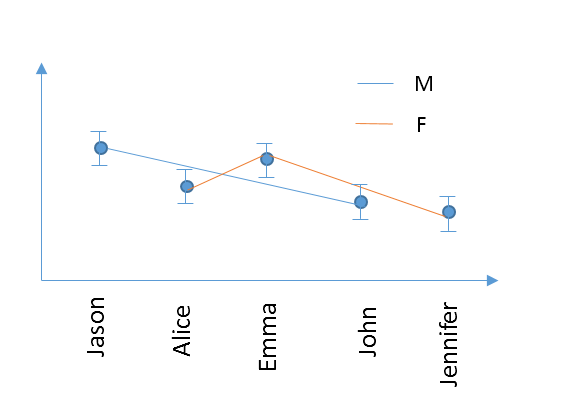Thanks a lot!

2 ACCEPTED SOLUTIONS

Accepted Solutions
Highlighted

## Re: how to plot an overlay variability chart

I wasn't sure about Score 1 and Score 2. I assumed that the two scores provides the range for your error bars. That means I stacked the Score columns first. For the Name column I turned on the Row Order Levels property so that JMP would plot them in the order they appear in the data table.

At the graph builder I drug the Name to the X-axis. I drug the Score column to the Y-axis. I changed the statistic to be the Mean with an Error Bar using the Range. I then drug the Gender column to the Overlay field and added the connector line. Results look like below. The JSL is below the graph.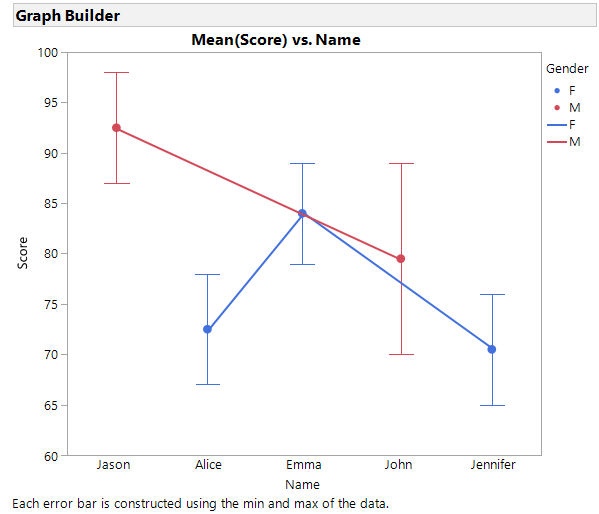``````Graph Builder(
Size( 528, 465 ),
Show Control Panel( 0 ),
Variables( X( :Name ), Y( :Score ), Overlay( :Gender ) ),
Elements(
Points(
X,
Y,
Legend( 5 ),
Summary Statistic( "Mean" ),
Error Bars( "Range" )
),
Line( X, Y, Legend( 6 ) )
),
SendToReport(
Dispatch(
{},
"400",
ScaleBox,
{Legend Model(
5,
Base( 0, 0, 0, Item ID( "F", 1 ) ),
Base( 1, 0, 0, Item ID( "M", 1 ) )
)}
),
Dispatch( {}, "Graph Builder", FrameBox, {Marker Size( 6 )} )
)
);
``````
Dan Obermiller
Highlighted

## Re: how to plot an overlay variability chart

You can create a new virtual column by right clicking on one of the variables in the column names in the Columns area, you can select Formula to create a new column in which you can concatenate the 3 column's values into one column, and then use that column to drag into the Page drop area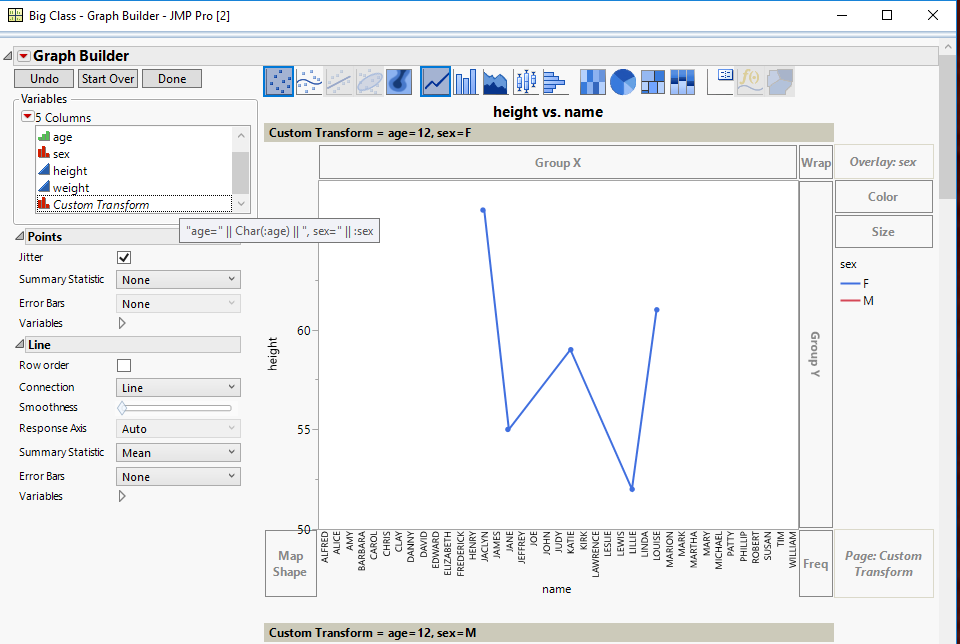Jim
16 REPLIES 16
Highlighted

## Re: how to plot an overlay variability chart

Graph Builder is what you want to use.  It is documented in the Essential Graphics document

Help==>Books==>Essential Graphing

The below graph was generated interactively, using the Big Class sample data table.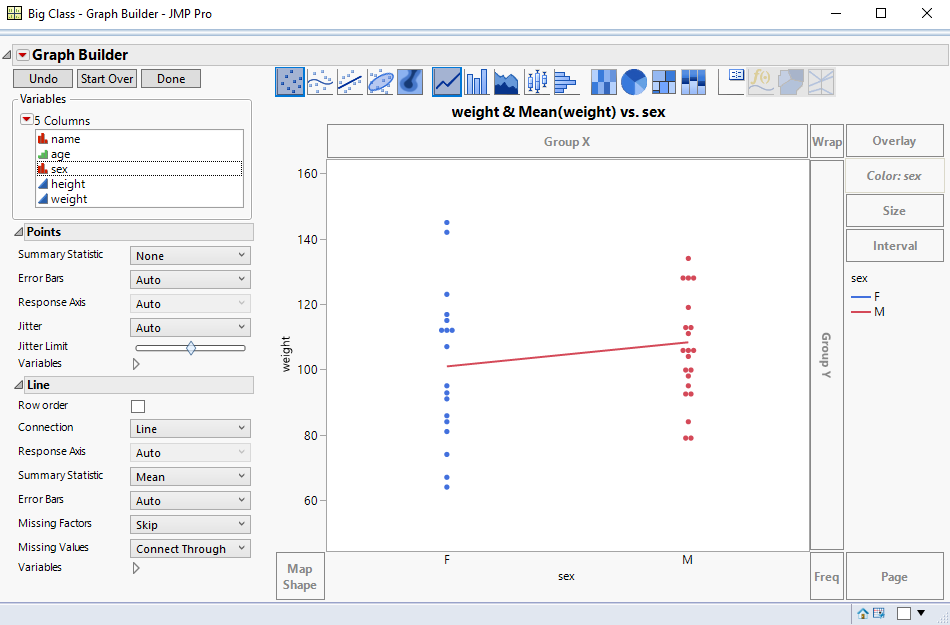Below is a script that also generates the above graph

``````Names Default To Here( 1 );
dt = Open( "\$SAMPLE_DATA/Big Class.jmp" );
Graph Builder(
Variables( X( :sex ), Y( :weight ), Color( :sex ) ),
Elements( Points( X, Y, Legend( 3 ) ), Line( X, Y, Legend( 4 ) ) ),
SendToReport(
Dispatch(
{},
"weight",
ScaleBox,
{Min( 44.3877551020408 ), Max( 164.387755102041 ),
Inc( 20 ), Minor Ticks( 0 )}
)
)
);``````
Jim
Highlighted

## Re: how to plot an overlay variability chart

Hi, you can use also

Analyze --> QUALITY and PROCESS --> VARIABILITY /ATTRIBUTE GAUGE CHART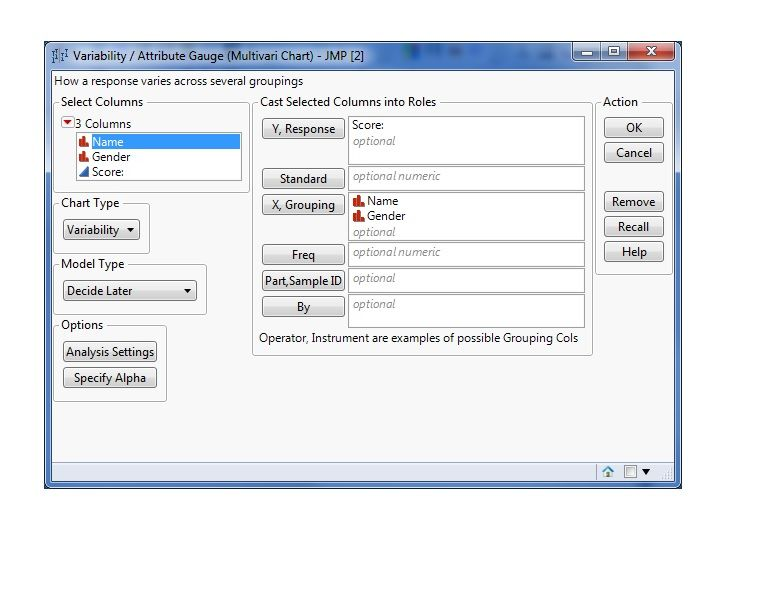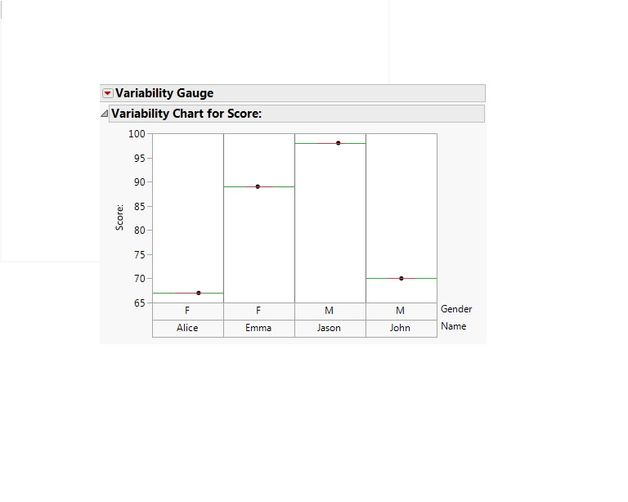Gianpaolo Polsinelli
Highlighted

## Re: how to plot an overlay variability chart

This is an extension of @txnelson and @gianpaolo suggestions.

• Add a sequence column call it rowid which is just the row number (this is needed for GraphBuilder)

Below are 3 examples

1. Variability which has only one connection color,
2. GraphBuilder allows different colored connectors but can be combersome if you want a true overlay with an X-axis like datetime
3. Bivariate with GroupBy allows colored connectors to match the group points, and here if you wanted x to be the original row order (like a Date axis) where the groups are spread, the graph will produce a true overlay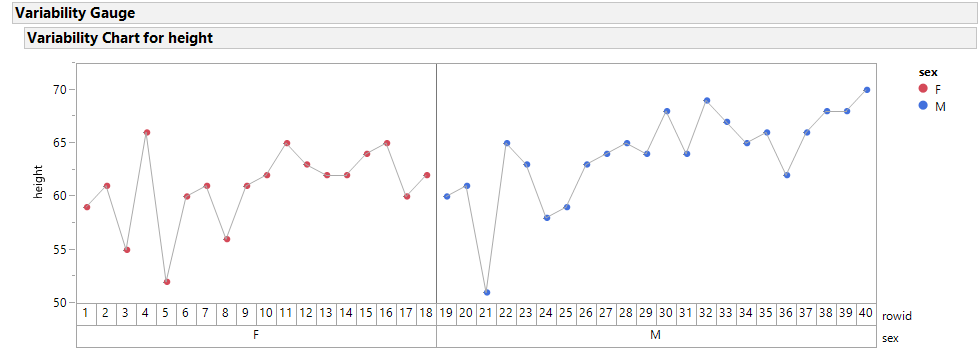Variability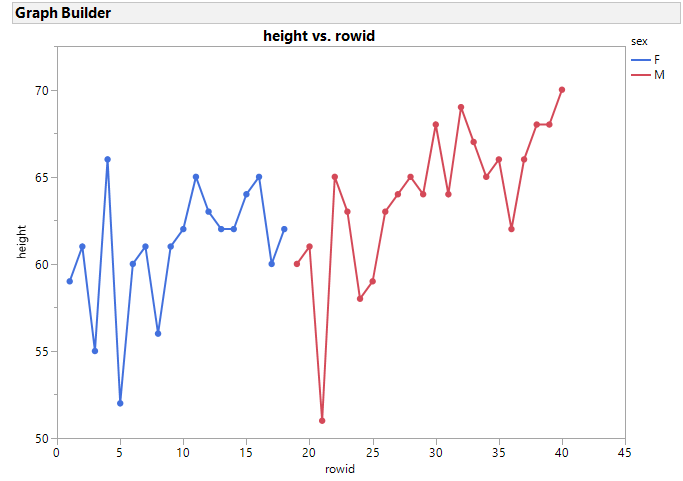GraphBuilder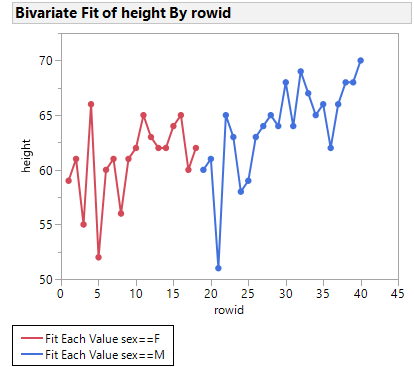Bivariate Group By :sex

Highlighted

## Re: how to plot an overlay variability chart

Thanks guys. Sorry, I didn't make it clear. Is there any possible way to plot like this?Highlighted

## Re: how to plot an overlay variability chart

I wasn't sure about Score 1 and Score 2. I assumed that the two scores provides the range for your error bars. That means I stacked the Score columns first. For the Name column I turned on the Row Order Levels property so that JMP would plot them in the order they appear in the data table.

At the graph builder I drug the Name to the X-axis. I drug the Score column to the Y-axis. I changed the statistic to be the Mean with an Error Bar using the Range. I then drug the Gender column to the Overlay field and added the connector line. Results look like below. The JSL is below the graph.``````Graph Builder(
Size( 528, 465 ),
Show Control Panel( 0 ),
Variables( X( :Name ), Y( :Score ), Overlay( :Gender ) ),
Elements(
Points(
X,
Y,
Legend( 5 ),
Summary Statistic( "Mean" ),
Error Bars( "Range" )
),
Line( X, Y, Legend( 6 ) )
),
SendToReport(
Dispatch(
{},
"400",
ScaleBox,
{Legend Model(
5,
Base( 0, 0, 0, Item ID( "F", 1 ) ),
Base( 1, 0, 0, Item ID( "M", 1 ) )
)}
),
Dispatch( {}, "Graph Builder", FrameBox, {Marker Size( 6 )} )
)
);
``````
Dan Obermiller
Highlighted

## Re: how to plot an overlay variability chart

Thanks Dan! It works! A further question. How I can I generate group plot in graph builder. Similar example as above

Name Gender Score1 Score2 Class

Jason  M 98 87 1

Alice F 67 78 1

John M 70 89 2

Emma F 89 79 2

Patrick 65 98 1

Jennifer 76 65 1

Dan 100 99 2

Wendy 78 84 2

Would like generate 2 plots by class, just like when you using group in varibility plot: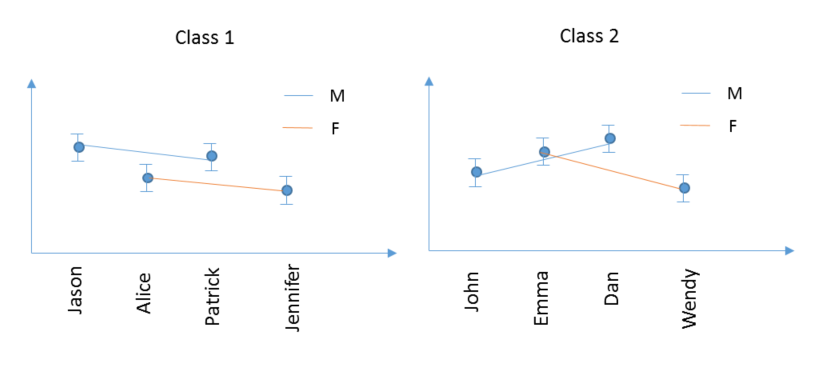Further more, if I have 3 varibales for grouping, such as class, area, school, so I would like to have 2x2x2=8 seperate plots, just like we use the group function in varibility plot.

Highlighted

## Re: how to plot an overlay variability chart

Just drag the Class variable to the Page drop area, or the Group By X drop area
Jim
Highlighted

## Re: how to plot an overlay variability chart

Thanks Jim. Is it possible to create 2 seperate plots instead squeezing all data in 1 plot? . If I have 3 varibales for grouping, such as class, area, school, so I would like to have 2x2x2=8 seperate plots, just like we use the group function in varibility plot.

Highlighted

## Re: how to plot an overlay variability chart

Dragging to the Page area will create the separate graphs, or you can click on the "Dialog" button, and go to a User Entry Dialog box that will let you specify all of your settings, and then select the Class variable for the BY selection
Jim
Article Labels

There are no labels assigned to this post.